# Common Core: 7th Grade Math : Interpret Products of Rational Numbers and Understand Properites of Operations: CCSS.Math.Content.7.NS.A.2a

## Example Questions

← Previous 1 3 4 5 6 7 8 9 10 11

### Example Question #1 : Interpret Products Of Rational Numbers And Understand Properites Of Operations: Ccss.Math.Content.7.Ns.A.2a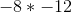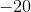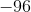Explanation:

When two negative values are multiplied, the answer becomes positive. Then, we multiply normally. We should get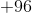or.

### Example Question #2 : Interpret Products Of Rational Numbers And Understand Properites Of Operations: Ccss.Math.Content.7.Ns.A.2a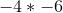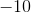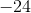Explanation:

When multiplying with negative numbers, we count the number of negative numbers. We have two so that means the answer is positive. So the product is### Example Question #3 : Interpret Products Of Rational Numbers And Understand Properites Of Operations: Ccss.Math.Content.7.Ns.A.2a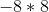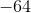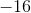Explanation:

When a positive number and a negative number is multiplied, the answer is negative. Just multiply normally. Answer is.

### Example Question #1 : Interpret Products Of Rational Numbers And Understand Properites Of Operations: Ccss.Math.Content.7.Ns.A.2a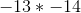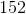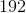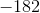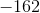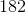Explanation:

When two negative numbers are multiplied, the answer is positive. Multiply normaly. Answer is.

### Example Question #5 : Interpret Products Of Rational Numbers And Understand Properites Of Operations: Ccss.Math.Content.7.Ns.A.2a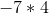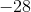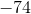Explanation:

When multiplying a negative number and a positive number, our answer is negative.

Multiply normally.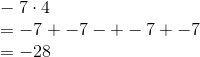Answer is.

### Example Question #51 : Negative Numbers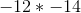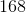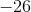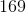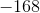Explanation:

When multiplying two negative numbers, our answer is positive.

Multiply normally. First multiply two by eight. Then multiply four and one.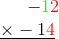Next, place a zero in the ones digit spot and multiply one by two followed by multiplying one by one.From here, add the two products together.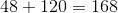Answer is.

### Example Question #1 : Interpret Products Of Rational Numbers And Understand Properites Of Operations: Ccss.Math.Content.7.Ns.A.2a

Solve: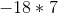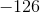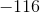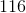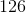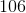Explanation:

When multiplying a negative number and a positive number, our answer is negative. Multiply normally. Answer is.

### Example Question #281 : Operations And Properties

Solve: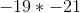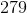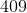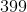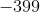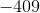Explanation:

When multiplying two negative numbers, the answer is positive. Then multiply normally. Answer is.

### Example Question #1 : Interpret Products Of Rational Numbers And Understand Properites Of Operations: Ccss.Math.Content.7.Ns.A.2a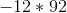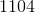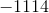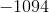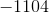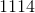Explanation:When multiplying with a negative number, our answer is negative.

Answer is### Example Question #10 : Interpret Products Of Rational Numbers And Understand Properites Of Operations: Ccss.Math.Content.7.Ns.A.2a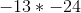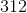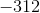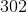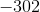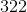Explanation:When multiplying with two negative numbers, our answer is positive.

Answer is← Previous 1 3 4 5 6 7 8 9 10 11

### All Common Core: 7th Grade Math Resources